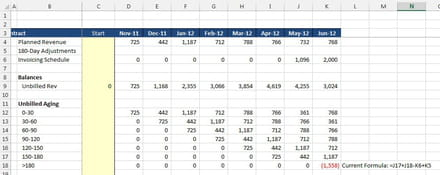# Apply a negative until 0 is reached in Excel

[Closed]
Posts
1
Registration date
Wednesday January 28, 2015
Status
Member
Last seen
January 28, 2015
-
Posts
122
Registration date
Tuesday August 31, 2010
Status
Contributor
Last seen
June 20, 2016
-
I am trying to create either a formula or a macro (which I do not know how to create) so that the following rule works: (1) If last row of data (ie cell K18) is < 0, then apply that negative number to the next oldest number in that same column until 0 or > is met; (2) Once the number is 0 or > then the cell where that was reached would have the remaining number; (3) All cells below that positive number would then be 0. I have attached a picture as an example: I would like to have the (1558) be applied to each value above it until it becomes 0 or > in the cells above it. In this example, the (1558) would be 0, the 1187 would be 0, and the 712 would then become 341...

I hope that made sense - it's hard to explain in words. I am trying to find a way to use nested If statements or a formula somehowThanks!

## 2 replies

Posts
122
Registration date
Tuesday August 31, 2010
Status
Contributor
Last seen
June 20, 2016
24
A single formula or IF's cannot do this as they can only interact with the cell they are located in.

A macro is the way to go.

This will do what you want for that column. Its not the prettiest piece of code and it very specific to your requirement but it does show what is possible.

```Sub rollup()
tot = Range("H18")
For n = 17 To 12 Step -1
newcell = Cells(n, 8).Value
If Abs(tot) > 0 Then
If Abs(tot) - newcell >= 0 Then
Cells(n, 8).Value = 0
tot = tot + newcell
Else
Cells(n, 8).Value = newcell - Abs(tot)
tot = 0
End If
Range("H18") = tot
End If

Next n
End Sub
```

A command button can be added to the worksheet to call it whenever you need an update to be done.
Awesome thanks. I was able to find the if function to work
Posts
122
Registration date
Tuesday August 31, 2010
Status
Contributor
Last seen
June 20, 2016
24
What was it?